Let's try another one:

 Let's graph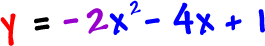, label 3 points (like we did before) and find where it crosses the x-axis (these are called "zeros"):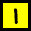Complete the square: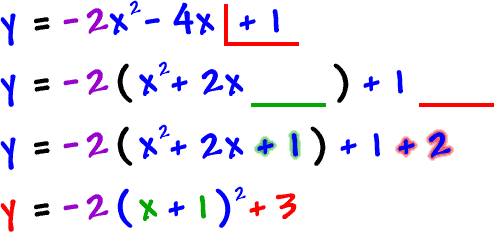Graph it and label three points: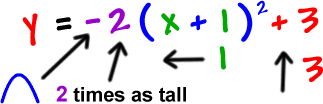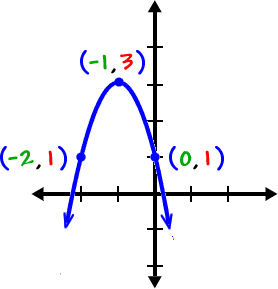Find the zeros:

*set it =0 and solve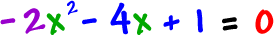We'll have to quadranate this one:Do these values make sense with our graph?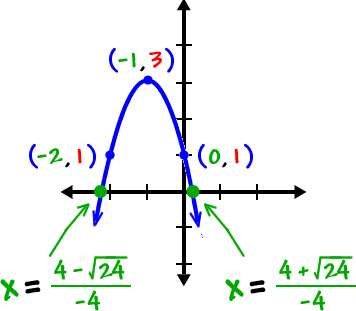YES!